# Density of Crystal - Solid State

Density of Crystal

If length of edge of unit cell  = l

Volume of unit cell =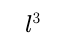Density of unit cell =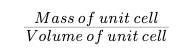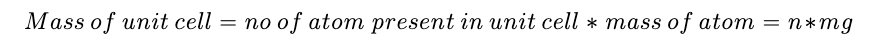Mass of one atom (m). =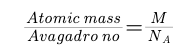Mass of unit cell  =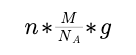Density of unit cell =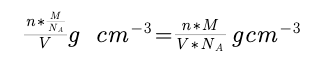Question :

Gold Au(197) crystellises in F.C.C whose crystal density is 19.3 gm/cm^3 .Calculate atomic radius of gold atom.

Solution :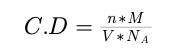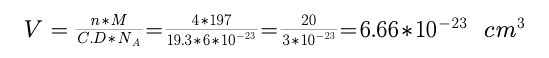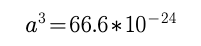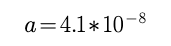In F.C.C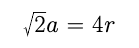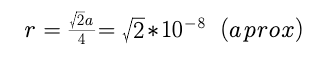Post By : Preeti Rai 12 Jun, 2020 366 views Chemistry